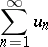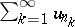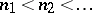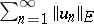# Unconditional convergence(*)
of elements of a linear spacein which the concept of a convergent sequence is defined is called unconditionally convergent if it converges after any rearrangement of its terms.
One approach to the study of unconditional convergence is the study of unconditionally convergent series in metric vector (or topological) spaces, . Thus, for the unconditional convergence of the series (*) of elements of a Banach space, it is necessary and sufficient for each partial series,, to be convergent  (the Orlicz theorem). Unconditional convergence of a numerical series is equivalent to its absolute convergence (cf. the Riemann theorem on the rearrangement of the terms of a series). In general, ifis a finite-dimensional normed space, unconditional convergence of a series is equivalent to convergence of the series. In an infinite-dimensional Banach space this is not valid.